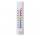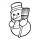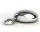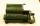In about 12 hours in North Dakota the temperature rose from -33 degrees farenheit to 50 degrees farenheit. By how much did the temperature change?

Result

t =  83 °F

Solution:Leave us a comment of this math problem and its solution (i.e. if it is still somewhat unclear...):Be the first to comment!Next similar math problems:

1. SubtractionTest what do you know about the subtraction of two numbers: a) make a difference if the minuend 4,307,288 and subtrahend is 472008 b) minuend is 4000 more than subtrahend. What's the difference? c) the difference is 38900 less than the minuend. What is th
2. The temperature 3The temperature is -12 degrees Fahrenheit. During the following 5 hours the temperature decrease 23 degrees Fahrenheit what is the temperature at 11 pm?
3. Temperature change 2The outside temperature changed -14F over 4 hours. If the temperature changed the same amount each hour, what was the change in temperature each hour?
4. Temperature 2Sunday's high temperature was 3 degrees higher than Saturday's. On Monday, the temperature fell 5 degrees, then rose 7 degrees on Tuesday and 4 more on Wednesday. Then it fell 17 degrees to a record low of 31 on Thursday. What was the temperature on Saturd
5. The temperature 2The temperature of Siberia fell13 degrees below 2/3 degrees centigrad. What is the temperature there now?
6. Thermometerthermometer showed -12 degrees Celsius at the morning then the temperature rises by 4 degrees and later again increased by 2 degrees at the evening has fallen by 5 degrees and then fall 3 degrees. What end temperature does the thermometer show?
7. FreezerTemperature is -8 degrees Celsius in the freezer. Outside temperature is 2 degrees Celsius. One minute took to change temperature by one degree Celsius if we open door. How many minutes the temperature in the freezer will rise?
8. This morningThis morning it was 80 degrees F outside the temperature increased 12 degrees F. What is the temperature now?
9. Degrees 2The temperature was 3°F and falls four degrees Fahrenheit. What is actually temperature?
10. Change in temperatureStarting temperature is 21°C, the highest temperature is 32°C. What is the change in temperature?
11. ThermometerThe thermometer showed -3°C at morning. Then temperature was increased by 1°C again increased by 1°C and then decreased by 1°C and then decreased by 4°C. Which terminal temperature thermometer shows?
12. On SundayOn Sunday the temperature reached a high of 38° above 0. That night it dropped to 23° below 0. What is the difference between the high and low temperatures for Sunday?
13. Melting pointThe melting point of mercury is -36°F and its boiling point is 672°F. What is the difference between the boiling point and the melting point?
14. Addition of Roman numbersAdded together and write as decimal number: LXVII + MLXIV
15. Flood waterFlood waters in some US village meant that the homes had to evacuate 364 people. 50 of them stayed at elementary schools, 59 them slept with their friends and others went to relatives. How many people have gone to relatives?
16. IntegersMay be the sum of two integers less than their difference?
17. SchoolThere are 150 pupils in grade 5 . 2/3 of it are female. By what fractions are the males?